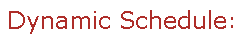Visit Minerva Class Schedule for course dates & times.

# COMP 611 Mathematical Tools for Computer Science (4 credits)

Offered by: Computer Science (Faculty of Science)

### Overview

Computer Science (Sci) : Introduction to mathematical concepts important across computer science, how to think mathematically, and how to write proofs. Proof techniques such as induction, contradiction, and monovariants; topics in combinatorics, graph theory, algebra, analysis, and probability; mathematical analysis of algorithms, data structures, and computational complexity. Emphasis on the mathematical explanations for useful concepts.

Terms: Fall 2022

Instructors: Rolnick, David (Fall)

• Restrictions: Not open to students who have majored in Mathematics or an equivalent subject, or have taken a proof-based math or computer science course within the previous two years.

• Not open to students who have taken COMP 761 when the topic was "Mathematical Tools for Computer Science".